## IMU原理

### 加速度计

$\mathbf{f}=\mathbf{a}-\mathbf{g}$

\mathbf{a}=\left.\frac{d^{2} \mathbf{r}}{d t^{2}}\right|_{i}=\ddot{\mathbf{r}}$$。 已知引力场向量为：$$\overline{\mathbf{g}}=\mathbf{g}+\Omega_{i e} \Omega_{i e} \mathbf{r}

$\mathbf{f}=\left.\frac{d^{2} \mathbf{r}}{d t^{2}}\right|_{i}-\mathbf{g}-\Omega_{i e} \Omega_{i e} \mathbf{r}$

### 陀螺仪

$\omega_{i b}^{b}=\omega_{i e}^{b}+\omega_{e n}^{b}+\omega_{n b}^{b}$

### 计算方法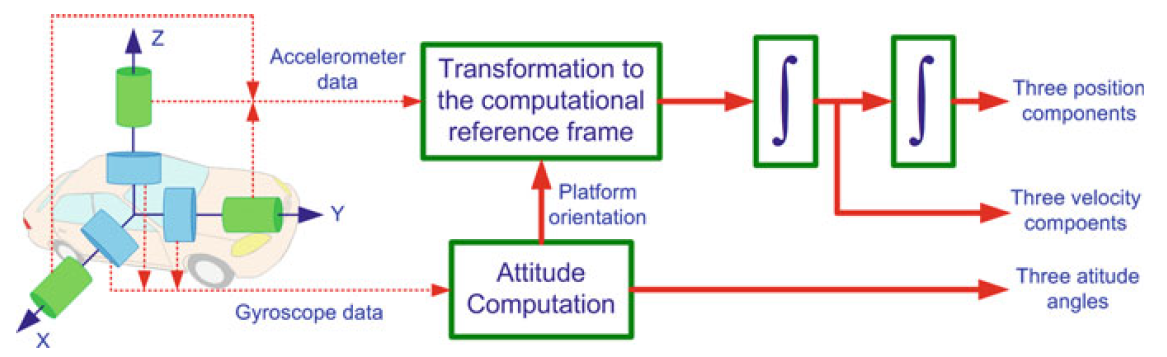## IMU误差

### 系统误差

#### Bias Offset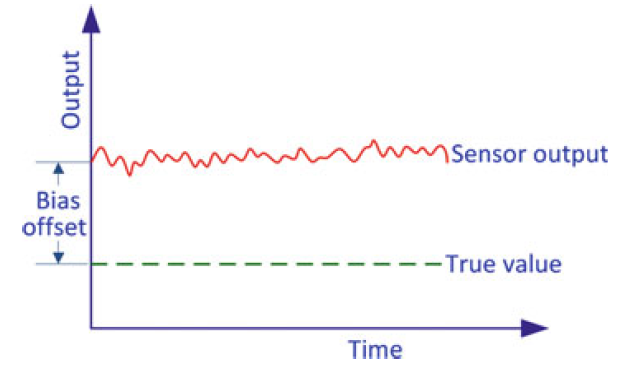#### Scale Factor Error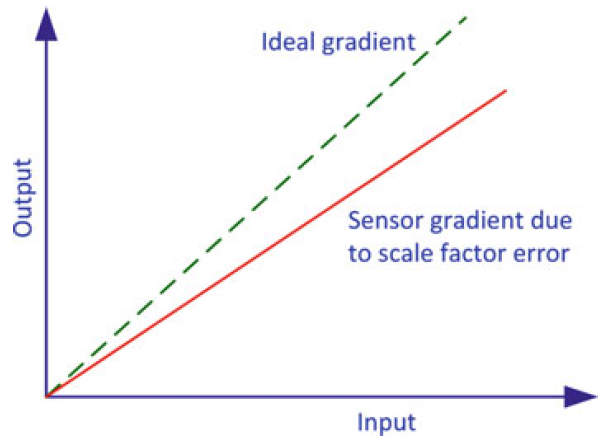#### Non-linearity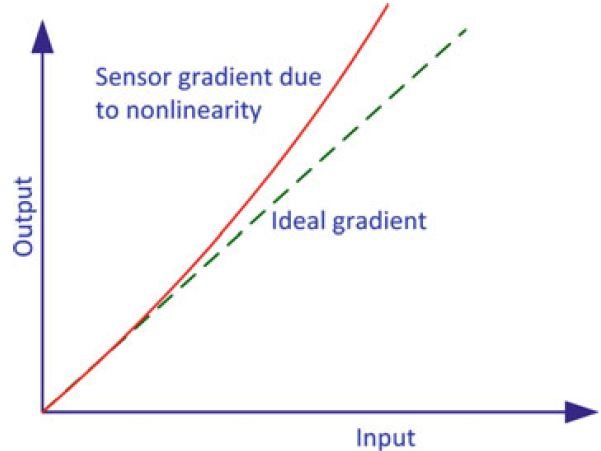#### 不对称与死区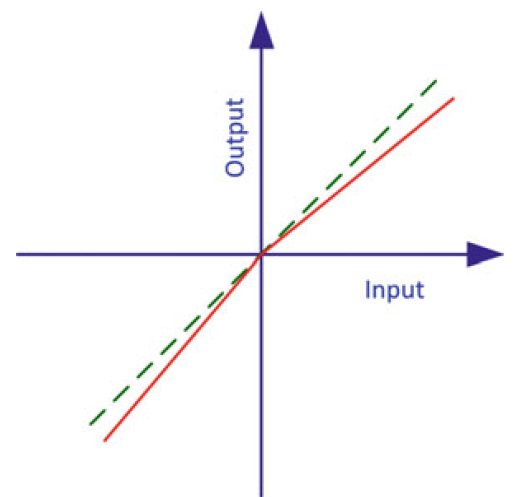#### Quantization Error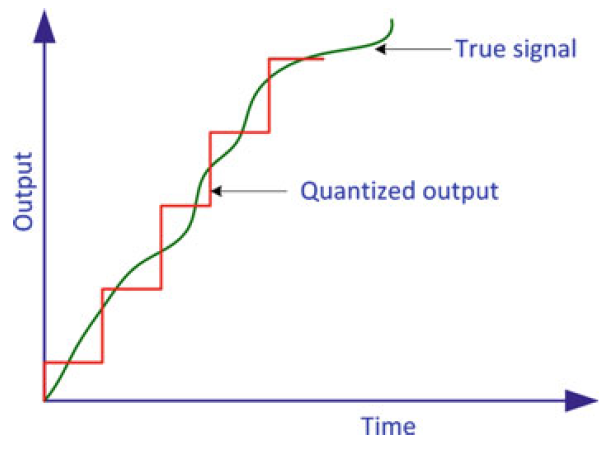#### Non-orthogonality Error 非正交误差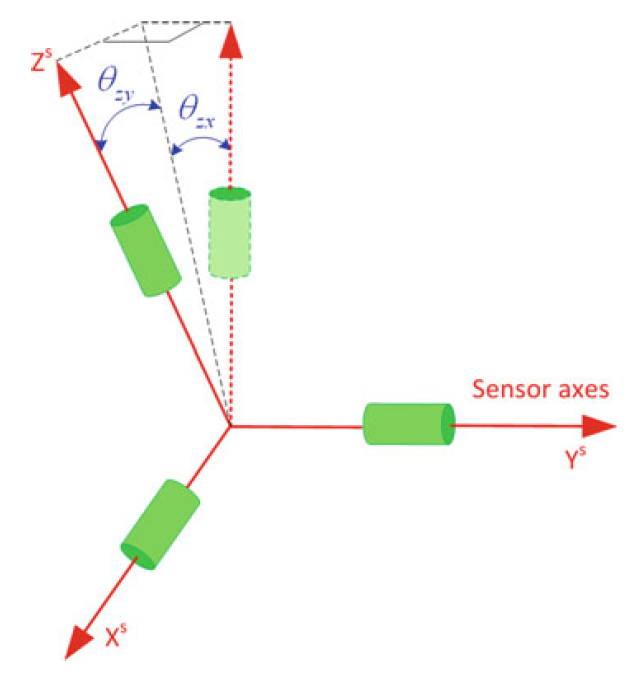#### Non-orthogonality Error 安装误差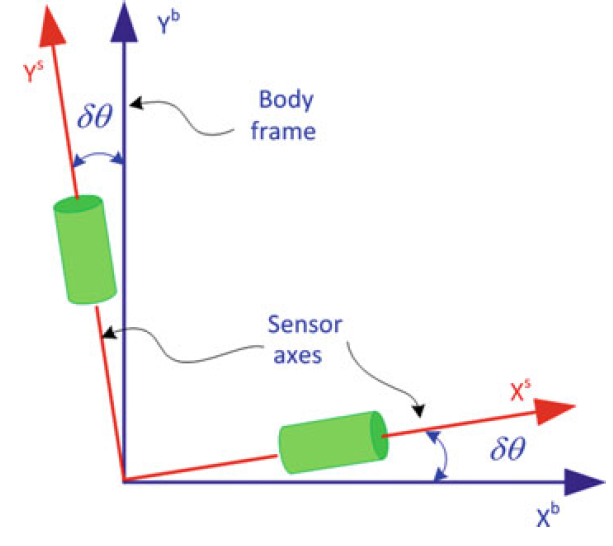### 随机误差

#### Run-to-Run Bias Offset

bias offset每次都变，不可重复。

#### Bias Drift/ Scale Factor Instability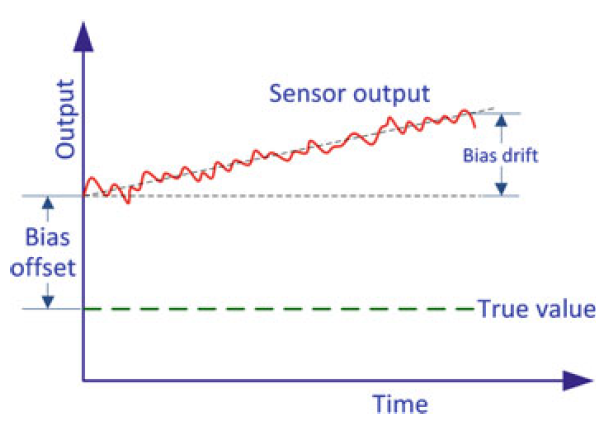#### white errors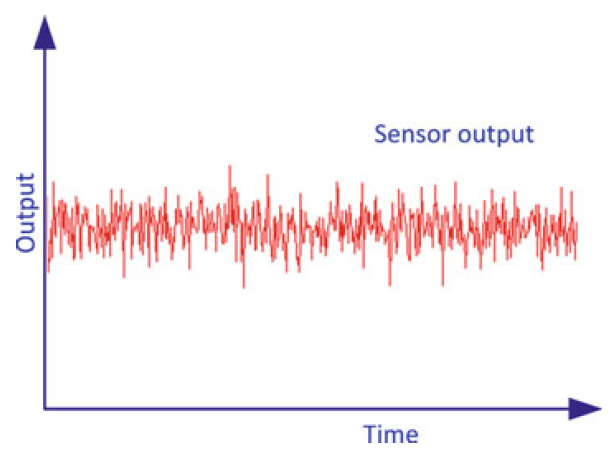## IMU实际测量模型

### 陀螺仪测量模型

$\tilde{\boldsymbol{\omega}}_{i b}^{b}=\boldsymbol{\omega}_{i b}^{b}+\mathbf{b}_{g}+S \boldsymbol{\omega}_{i b}^{b}+N \boldsymbol{\omega}_{i b}^{b}+\boldsymbol{\varepsilon}_{g}$

$\tilde{\omega}_{i b}^{b} \quad \text { 是陀螺仪角速度测量值 }(\mathrm{deg} / \mathrm{h})$

$\boldsymbol{\omega}_{i b}^{b} \quad \text { 是角速度真值 }(\mathrm{deg} / \mathrm{h})$

$\mathbf{b}_{g} \quad \text { bias }(\mathrm{deg} / \mathrm{h})$

$S_{g} \quad scale\_factor$

$N_{g} \quad \text{代表非正交误差的矩阵}$

$\boldsymbol{\varepsilon}_{g} \quad \text { 陀螺仪噪声 }(\mathrm{deg} / \mathrm{h})$

$N_{g}=\left[\begin{array}{ccc}1 & \theta_{g, x y} & \theta_{g, x z} \\ \theta_{g, y x} & 1 & \theta_{g, y z} \\ \theta_{g, z x} & \theta_{g, z y} & 1\end{array}\right]$

$S_{g}=\left[\begin{array}{ccc}s_{g, x} & 0 & 0 \\ 0 & s_{g, y} & 0 \\ 0 & 0 & s_{g, z}\end{array}\right]$

### 加速度计测量模型

$\tilde{\mathbf{f}}^{b}=\mathbf{f}^{\mathbf{b}}+\mathbf{b}_{\mathbf{a}}+\mathbf{S}_{1} \mathbf{f}+\mathbf{S}_{\mathbf{2}} \mathbf{f}^{2}+\mathbf{N}_{\mathbf{a}} \mathbf{f}+\delta \mathbf{g}+\mathbf{\varepsilon}_{\mathbf{a}}$

$\mathbf{f}^{b} 加速度计加速度测量值$

$\mathbf{b}_{a} 加速度真值$

$S_{1} 线性scale\_factor$

$S_{2} 非线性scale\_factor$

$N_{a} 代表非正交矩阵的误差$

$\delta \mathbf{g} 异常的重力向量（如与理论重力的偏离）$

$\boldsymbol{\varepsilon}_{a} 加速度计噪声$

$N_{a}=\left[\begin{array}{ccc}1 & \theta_{a, x y} & \theta_{a, x z} \\ \theta_{a, y x} & 1 & \theta_{a, y z} \\ \theta_{a, z x} & \theta_{a, z y} & 1\end{array}\right]$

$S_{1}=\left[\begin{array}{ccc}s_{1, x} & 0 & 0 \\ 0 & s_{1, y} & 0 \\ 0 & 0 & s_{1, z}\end{array}\right]$

$S_{2}=\left[\begin{array}{ccc}s_{2, x} & 0 & 0 \\ 0 & s_{2, y} & 0 \\ 0 & 0 & s_{2, z}\end{array}\right]$

## IMU初始化与校准

### 姿态校准

1. 先通过加速度计获取pitch和roll

\begin{aligned} \mathbf{f}^{b} &=R_{l}^{b}\left(-\mathbf{g}^{l}\right) =\left(R_{b}^{l}\right)^{T}\left(-\mathbf{g}^{l}\right) \end{aligned}

$\mathbf{g}^{l}=\left[\begin{array}{lll}0 & 0 & -g\end{array}\right]^{T}$

$\mathbf{f}^{b} = \left[\begin{array}{l}f_{x} \\ f_{y} \\ f_{z}\end{array}\right]$

1. 再通过陀螺仪获取yaw。

$\omega_{i b}^{b}=\omega_{i e}^{b}+\omega_{e b}^{b}$

\begin{aligned} \omega_{i b}^{b} &=\omega_{i e}^{b}+0 \\ \omega_{i b}^{b} &=R_{e}^{b} \omega_{i e}^{e} \\ \omega_{i b}^{b} &=R_{l}^{b} R_{e}^{l} \omega_{i e}^{e} \\ \omega_{i b}^{b} &=\left(R_{b}^{l}\right)^{T}\left(R_{l}^{e}\right)^{T} \omega_{i e}^{e} \end{aligned}

$\omega_{i e}^{e}=\left[\begin{array}{lll}0 & 0 & \omega_{e}\end{array}\right]^{T}$

$\omega_{e}$ 大约为15.04 deg/h。

//TODO

//TODO

## 参考

 Noureldin A, Karamat T B, Georgy J. Fundamentals of inertial navigation, satellite-based positioning and their integration[J]. 2013.